# FQRestore

Author V. C. Mohan

FQRestore function operates in frequency domain and requires for avisynth FFTw3 32 bit dll or for avisynth+ FFTw3 64 bit dll, to be in the path.

Given the type and estimate of blur present, FQRestore function tries to deblur the image.

In case of motion blur the line is assumed to be symmetric about origin, the end coordinates of blur line will be -x, -y and x,y. In case of out of focus blur the x coordinate is the radius of blur.

While all 3 color planes of RGB formats are processed, in case of YUY2 and Planar only Luma (Y) is restored.

Inverting in frequency domain can lead to instabilities and to counter this white noise is added, which while stabilising reduces effectiveness. The radius in spatial domain of the inversion operator can be controlled. This can provide some desirable reduction of ringing. These two operators have effect on processed amplitude of the image. Therefore it is essential to experiment exhaustively to arrive at optimum scaling parameter for any particular situation.

For some situations for a particular set of parameters the result may be highly focussing in parts and in other parts amplitudes may be zero. One such condition is given in the examples. One must be careful about such situations.

For blur amounts over 3 ringing may be noticeable especially due to edges of image. Cropping result may be a solution.

 Description Name Type Limits Default Input clip clip must have the fields separated none Whether blur is linear as in motion blur or out of focus type line boolean true for motion blur, false for focus true white noise to add wn float 0.0001 to 0.99 0.05 blur line right end x coordinate or radius of focus blur. Line assumed symmetric about origin x integer 2 to 1/8 frame width 6 blur line right end y coordinate. Line assumed symmetric about origin y integer - 1/8th to 1/8th frame height 2 scaling to be applied post processing scale float 0.01 to 1000000 1.0 Radius of filter in spatial domain, as %age of smaller of the frame dimensions fr float 0.01 to 39.99 10

#Usage examples. These also demonstrate the artifacts produced and need to select proper scale value:-
```### effect of amount of motion blur
## using FQBLUR as input
FQRestore()
FQRestore(line = true,x = 2, y = 2fr = 20,wn = 0.05,scale = 0.45)
FQRestore(line = true,x = 8, y = 4,fr = 30,wn = 0.05,scale = 0.375)

```

effect of amount of motion blur
below are results. Left is blurred image. Right restored image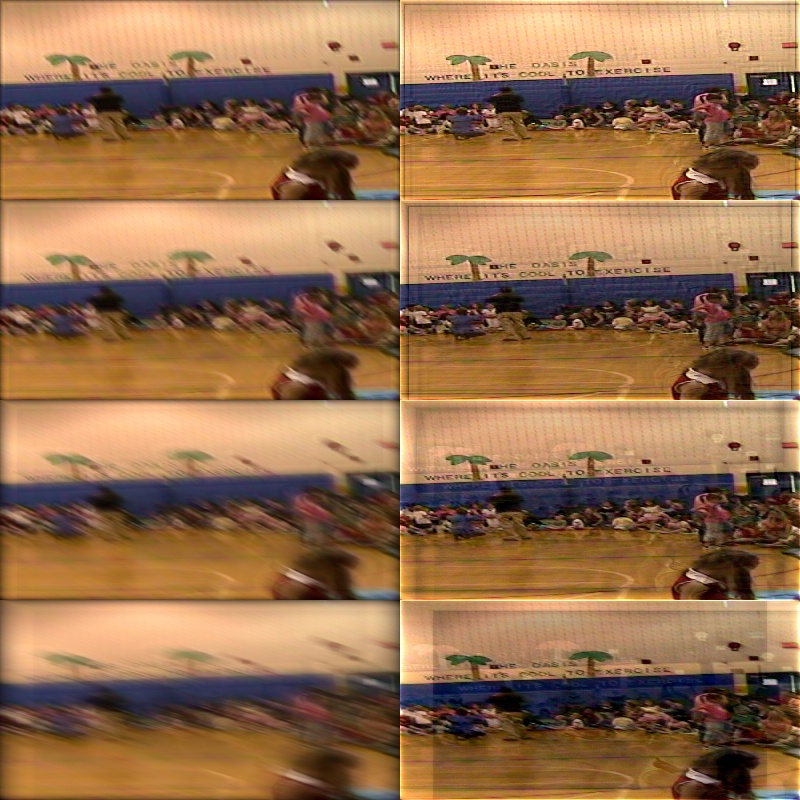```###effect of filter radius
FQBlur( line = false, x=8,y = 0)
FQRestore(line = false,x = 8, y = 0,fr = 20,wn = 0.08,scale = 0.45)
FQRestore(line = false,x = 8, y = 0,fr = 39,wn = 0.08,scale = 0.45)
FQRestore(fqb8,line = false,x = 8, y = 0,fr = 60,wn = 0.08,scale = 0.45)
FR8 = FQRestore(fqb8,line = false,x = 8, y = 0,fr = 80,wn = 0.08,scale = 0.45)
st1 = stackvertical(last, fr2,fr4)
st2 = stackvertical(fqb8, fr6,fr8)
stackhorizontal(st1,st2)```

below are results. top Left is original. top Right blurred image used as input for all. note amplitude variation.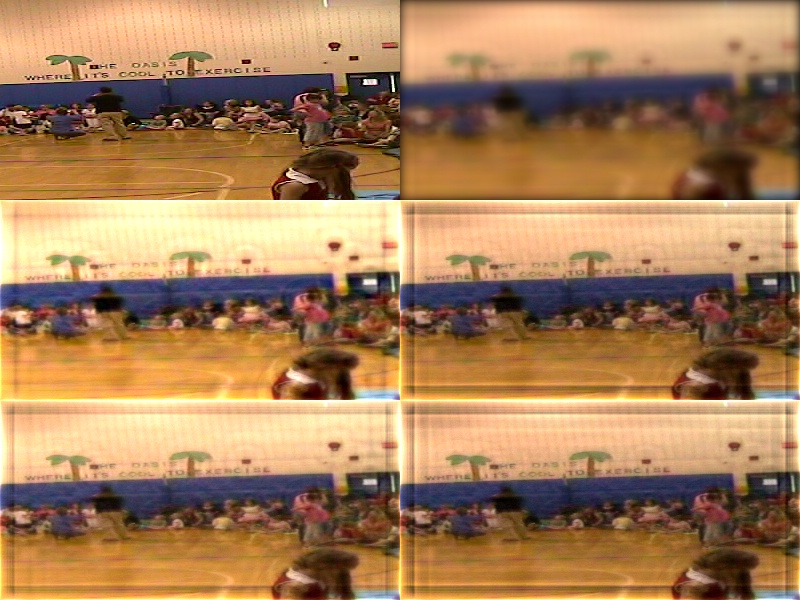```# Degree of defocus of input
fqb2 = FQBlur( line = false, x=2,y = 0)
fqb4 = FQBlur( line = false, x=4,y = 0)
fqb8 = FQBlur( line = false, x=8,y = 0)
fqb16 = FQBlur( line = false, x=16,y = 0)

FR2 = FQRestore(fqb2,line = false,x = 2, y = 0,fr = 20,wn = 0.08,scale = 0.45)
FR4 = FQRestore(fqb4,line = false,x = 4, y = 0,fr = 20,wn = 0.08,scale = 0.45)
FR8 = FQRestore(fqb8,line = false,x = 8, y = 0,fr = 20,wn = 0.08,scale = 0.45)
FR16 = FQRestore(fqb16,line = false,x = 16, y = 0,fr = 20,wn = 0.08,scale = 0.45)

st1 = stackvertical(fqb2,fqb4,fqb8, fqb16)
st2 = stackvertical(fr2, fr4,fr8, fr16)
stackhorizontal(st1,st2)```

Degree of defocus of input
Left input Right restored image. scale was kept constant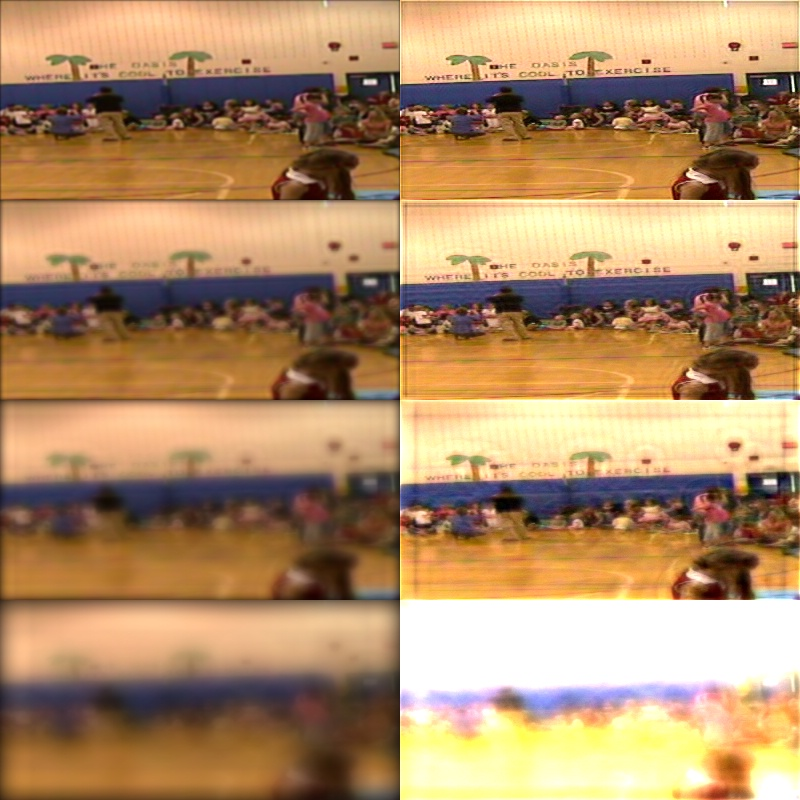```### effect of white noise value wn
wn
Top left Original, Top right blurred input, scale kept constant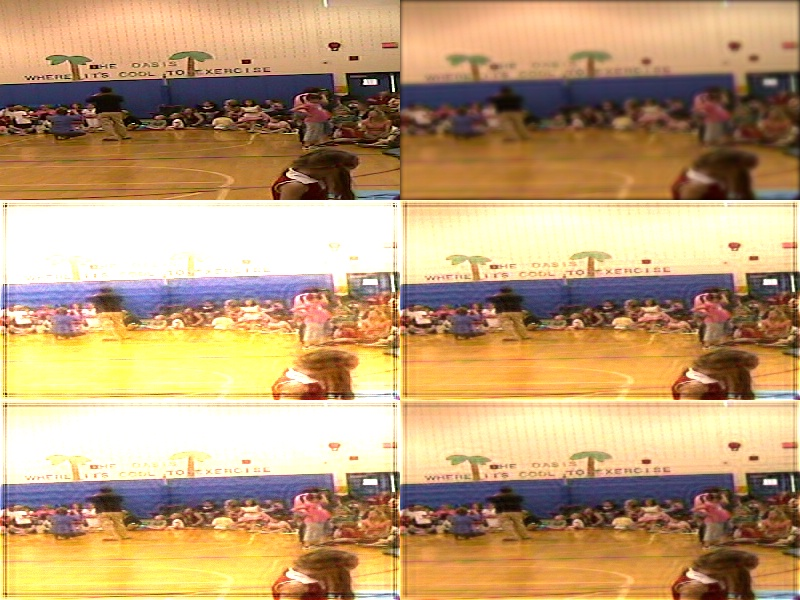### example of an unstable(?) situation

unstable(?) situation. Top large focus blurred input.Lower 3 processed
with very small difference in parameter fr (Filter radius). Note to control amplitude
scale value needed was highest for the central one.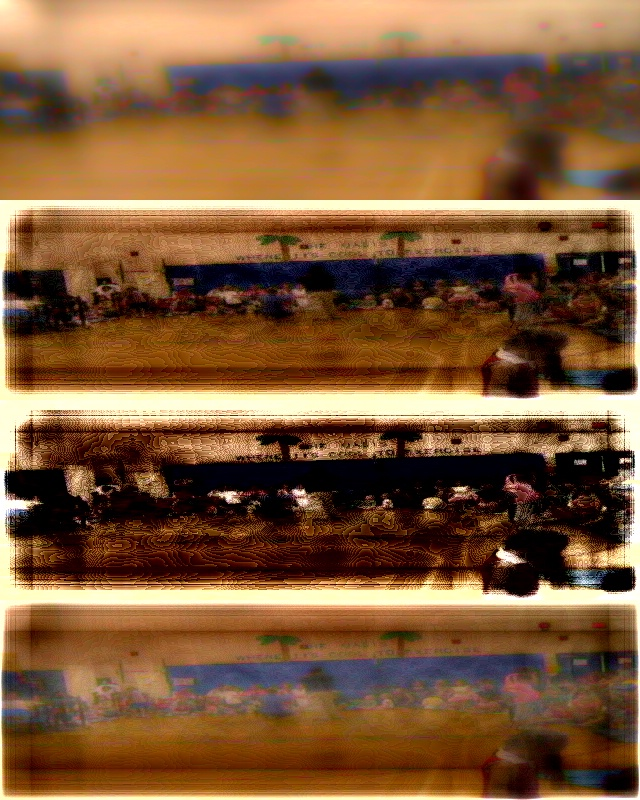<

To my index pageTo Avisynth

```